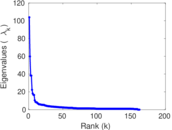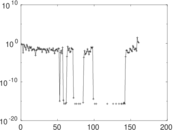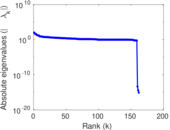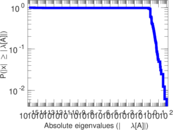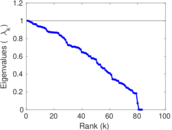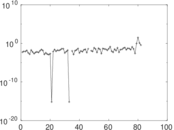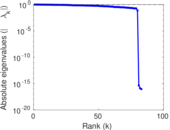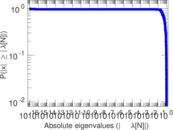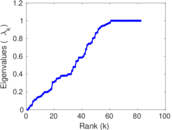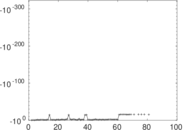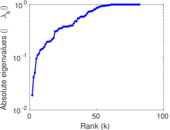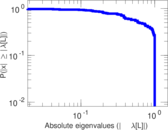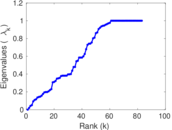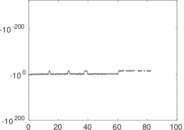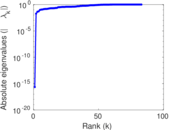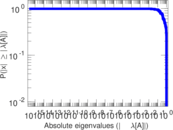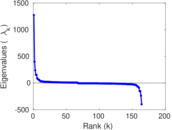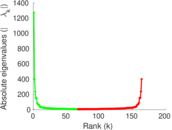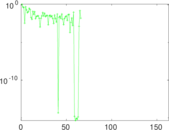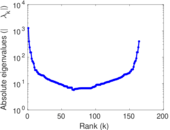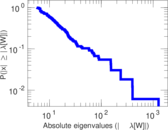# Wiktionary edits (ti)

This is the bipartite edit network of the Tigrinya Wiktionary. It contains users and pages from the Tigrinya Wiktionary, connected by edit events. Each edge represents an edit. The dataset includes the timestamp of each edit.

 Code `mti` Internal name `edit-tiwiktionary` Name Wiktionary edits (ti) Data source http://dumps.wikimedia.org/ AvailabilityDataset is available for download Consistency checkDataset passed all tests Category Authorship network Dataset timestamp 2017-10-20 Node meaning User, article Edge meaning Edit Network formatBipartite, undirected Edge typeUnweighted, multiple edges Temporal dataEdges are annotated with timestamps

## Statistics

 Size n = 1,282 Left size n1 = 164 Right size n2 = 1,118 Volume m = 3,148 Unique edge count m̿ = 1,864 Wedge count s = 149,277 Claw count z = 12,467,295 Cross count x = 860,094,700 Square count q = 109,504 4-Tour count T4 = 1,477,176 Maximum degree dmax = 849 Maximum left degree d1max = 849 Maximum right degree d2max = 57 Average degree d = 4.911 08 Average left degree d1 = 19.195 1 Average right degree d2 = 2.815 74 Fill p = 0.010 166 2 Average edge multiplicity m̃ = 1.688 84 Size of LCC N = 714 Diameter δ = 11 50-Percentile effective diameter δ0.5 = 3.324 90 90-Percentile effective diameter δ0.9 = 5.582 61 Median distance δM = 4 Mean distance δm = 3.812 54 Gini coefficient G = 0.730 025 Balanced inequality ratio P = 0.211 404 Left balanced inequality ratio P1 = 0.128 653 Right balanced inequality ratio P2 = 0.284 943 Relative edge distribution entropy Her = 0.804 829 Power law exponent γ = 2.656 55 Tail power law exponent γt = 2.361 00 Tail power law exponent with p γ3 = 2.361 00 p-value p = 0.047 000 0 Left tail power law exponent with p γ3,1 = 1.711 00 Left p-value p1 = 0.253 000 Right tail power law exponent with p γ3,2 = 3.581 00 Right p-value p2 = 0.008 000 00 Degree assortativity ρ = −0.031 195 2 Degree assortativity p-value pρ = 0.178 222 Algebraic connectivity a = 0.018 912 0 Spectral separation |λ1[A] / λ2[A]| = 1.735 56 Controllability C = 696 Relative controllability Cr = 0.686 391

## Plots

### Fruchterman–Reingold graph drawing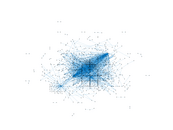### Degree distribution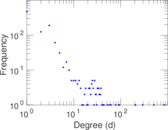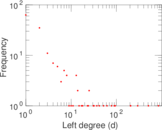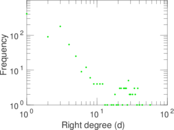### Cumulative degree distribution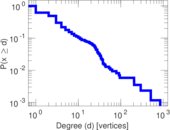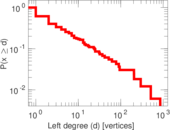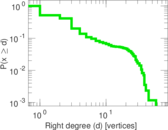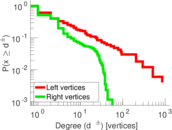### Lorenz curve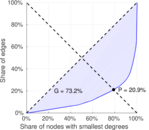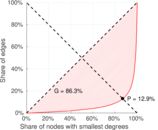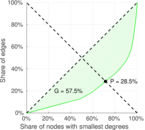### Spectral distribution of the adjacency matrix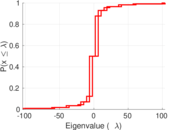### Spectral distribution of the normalized adjacency matrix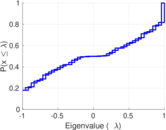### Spectral distribution of the Laplacian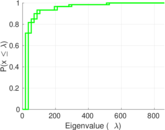### Spectral graph drawing based on the adjacency matrix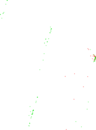### Spectral graph drawing based on the Laplacian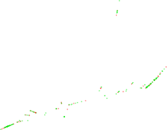### Spectral graph drawing based on the normalized adjacency matrix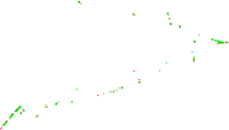### Degree assortativity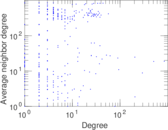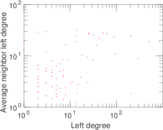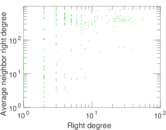### Zipf plot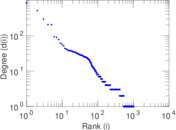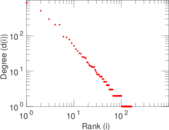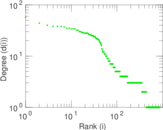### Hop distribution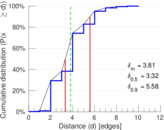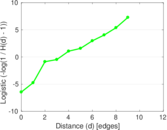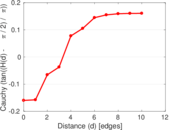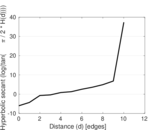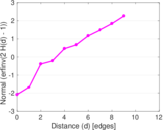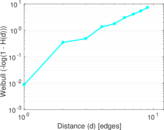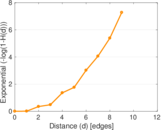### Double Laplacian graph drawing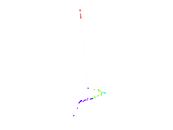### Delaunay graph drawing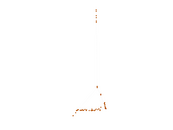### Edge weight/multiplicity distribution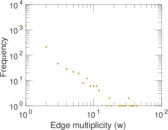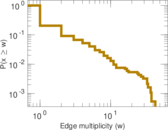### Temporal distribution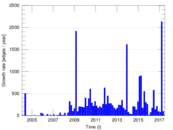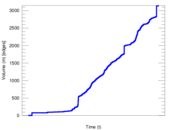### Temporal hop distribution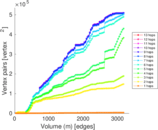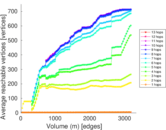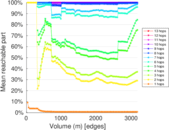### Diameter/density evolution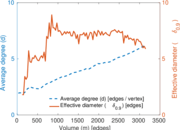### Matrix decompositions plots